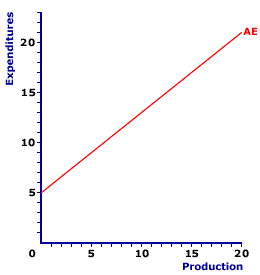Saturday  October 1, 2022
 AmosWEB means Economics with a Touch of Whimsy!ELASTICITY ALTERNATIVES, SUPPLY: The price elasticity of supply can fall into one of five categories--perfectly elastic, relatively elastic, unit elastic, relatively inelastic, and perfectly inelastic--based on the coefficient of elasticity. This table summarizes the five alternatives. These five elasticity alternatives form a continuum ranging from perfectly elastic at one end to perfectly inelastic at the other. The "middle" of this continuum is occupied by unit elastic. in that the "unit" and the two "perfectly" are really borders, boundaries, and endpoints, most of the real world action involving the price elasticity of supply takes place in the two "relatively" alternatives--relatively elastic and relatively inelastic.SLOPE, AGGREGATE EXPENDITURES LINE:

The positive slope of the aggregate expenditures line is the sum of the marginal propensity to consume (MPC), marginal propensity to invest (MPI), and marginal propensity for government purchases (MPG), less the marginal propensity to import (MPM). This slope is greater than zero but less than one, reflecting induced expenditures by the four macroeconomic sectors (household, business, government, and foreign). The slope of the aggregate expenditures line determines the magnitude of the multiplier process.
Aggregate Expenditures LineThe aggregate expenditures line, which embodies the key Keynesian principle of effective demand, shows the relation between aggregate expenditures and the actual level of aggregate income or production in the domestic economy. The income and production measures commonly used are national income and gross domestic product.

The two basic types of expenditures--induced and autonomous--are indicated by the aggregate expenditures line.

A representative aggregate expenditures line is presented in the exhibit to the right. This red line, labeled AE in the exhibit, is positively sloped, indicating that greater levels of income generate greater aggregate expenditures by the four sector. This positive relation is primarily based on the Keynesian psychological law indicating that consumption expenditures are induced by household income. However, this positive slope is reinforced and augmented by induce investment, government purchases, and net exports.

The slope of the aggregate expenditures line presented here is positive, but less than one. Click the [Slope] button to illustrate. In fact, the slope of the aggregate expenditures line is numerically based on the marginal expenditure propensities for any of the induced expenditures included in the aggregate expenditures line. For a "complete" four-sector aggregate expenditures line, the slope is equal to the sum of the marginal propensity to consume (MPC), marginal propensity to invest (MPI), and marginal propensity for government purchases (MPG), less the marginal propensity to import (MPM). If induced taxes are also included, then the slope is adjusted for the marginal tax rate.

To illustrate the connection between slope and the marginal expenditure propensities, consider the equation for the slope of the aggregate expenditures line, specified as the "rise" over the "run." The rise is the change in aggregate expenditures measured on the vertical axis and the run is the change in income (or production) measured on the horizontal axis.

 slope = riserun = change in aggregate expenditureschange in income
However, for any induced movement on the aggregate expenditures line, the change in aggregate expenditures includes changes in consumption expenditures, investment expenditures, government purchases, or net exports. The slope of the aggregate expenditures line can thus be specified as based on each of these expenditures.
 slope = change in (consumption + investment + government purchases + net exports)change in income
This can be rewritten as:
 slope = change inconsumptionchange inincome + change ininvestmentchange inincome + change ingovernment purchaseschange inincome + change innet exportschange inincome
Because the induced change in net exports is attributable totally to the induced change in imports and because imports are subtracted for exports to derive net exports, the change in net exports is equal to the negative change in imports. The slope can be rewritten as:
 slope = change inconsumptionchange inincome + change ininvestmentchange inincome + change ingovernment purchaseschange inincome - change inimportschange inincome
Each of these terms is a corresponding marginal propensity.
 slope = marginal propensityto consume + marginal propensityto invest + marginal propensityfor government purchases - marginal propensityto import
Or, using standard abbreviations that are both symbolic and a little easier to remember.
 slope = MPC + MPI + MPG - MPM
Because each of these marginals is the slope of the corresponding expenditure line (consumption line, investment line, government purchases line, and net exports line), the slope of the aggregate expenditures line is the summation of the slopes of the lines for each of the four aggregate expenditures.

The positive slope of the aggregate expenditures line reflects induced expenditures, which are aggregate expenditures that depend on the level of income or production. If the aggregate economy has more income, then the four macroeconomic sectors are induced to undertake additional expenditures. Of course, a drop in aggregate income or production induces the sectors to reduce expenditures.

 <= SLOPE, AGGREGATE DEMAND CURVE SLOPE, CONSUMPTION LINE =>Recommended Citation:

SLOPE, AGGREGATE EXPENDITURES LINE, AmosWEB Encyclonomic WEB*pedia, http://www.AmosWEB.com, AmosWEB LLC, 2000-2022. [Accessed: October 1, 2022].

Check Out These Related Terms...

Or For A Little Background...

And For Further Study...
Search Again?YELLOW CHIPPEROON[What's This?] Today, you are likely to spend a great deal of time at a crowded estate auction looking to buy either a set of luggage with wheels or a birthday gift for your aunt. Be on the lookout for door-to-door salesmen.Your Complete ScopeOkun's Law posits that the unemployment rate increases by 1% for every 2% gap between real GDP and full-employment real GDP."The truth is not for all men, but only for those who seek it. "-- Ayn Rand, writerACVActual Cash ValueA PEDestrian's Guide Xtra CreditTell us what you think about AmosWEB. Like what you see? Have suggestions for improvements? Let us know. Click the User Feedback link.| | | | | | | | | | |
| | | |

Thanks for visiting AmosWEB